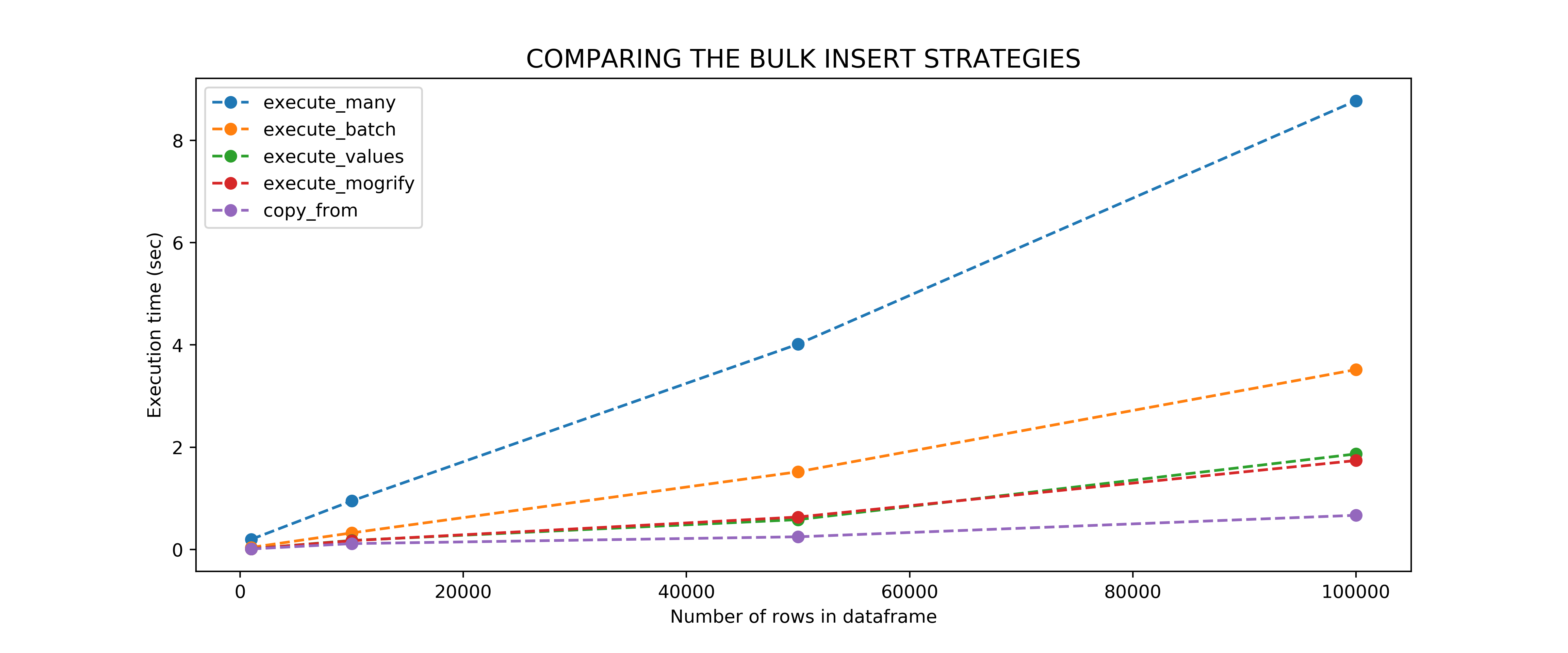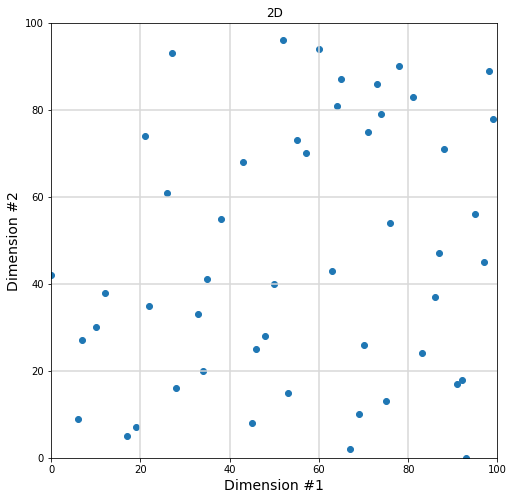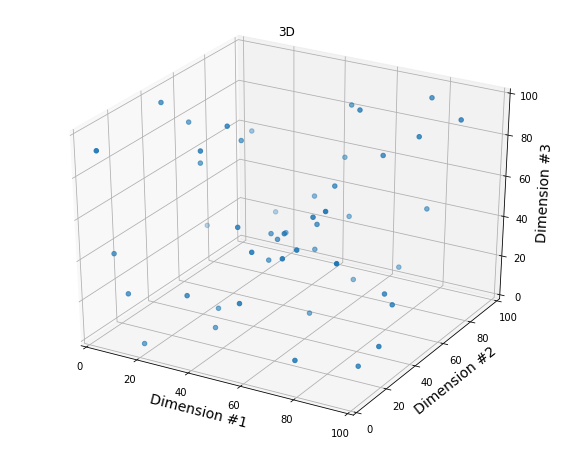# Python

## Pandas to PostgreSQL using Psycopg2: Bulk Insert Using execute_values()

As you can see at the end of my benchmark post, the 3 acceptable ways (performance wise) to do a bulk insert in Psycopg2 are

• execute_values()
• execute_mogrify()
• copy_from()

This post provides an end-to-end working code for the execute_values() option.

### Step 1: Specify the Connection Parameters

```# Here you want to change your database, username & password according to your own values
param_dic = {
"host"      : "localhost",
"database"  : "globaldata",
"user"      : "myuser",
}```

### Step 2: Helper Functions

```def connect(params_dic):
""" Connect to the PostgreSQL database server """
conn = None
try:
# connect to the PostgreSQL server
print('Connecting to the PostgreSQL database...')
conn = psycopg2.connect(**params_dic)
except (Exception, psycopg2.DatabaseError) as error:
print(error)
sys.exit(1)
print("Connection successful")
return conn

def execute_values(conn, df, table):
"""
Using psycopg2.extras.execute_values() to insert the dataframe
"""
# Create a list of tupples from the dataframe values
tuples = [tuple(x) for x in df.to_numpy()]
# Comma-separated dataframe columns
cols = ','.join(list(df.columns))
# SQL quert to execute
query  = "INSERT INTO %s(%s) VALUES %%s" % (table, cols)
cursor = conn.cursor()
try:
extras.execute_values(cursor, query, tuples)
conn.commit()
except (Exception, psycopg2.DatabaseError) as error:
print("Error: %s" % error)
conn.rollback()
cursor.close()
return 1
print("execute_values() done")
cursor.close()

```

### Step 3: Main Code

```# Read the csv file

# Connect to the database
conn = connect(param_dic)

# Run the execute_many strategy
execute_values(conn, df, 'MonthlyTemp')

# Close the connection
conn.close()
```

For a fully functioning tutorial on how to replicate this, please refer to my Jupyter notebook  and Python script on GitHub.

## Pandas to PostgreSQL using Psycopg2: Bulk Insert Performance Benchmark

If you have ever tried to insert a relatively large dataframe into a PostgreSQL table, you know that single inserts are to be avoided at all costs because of how long they take to execute.  There are multiple ways to do bulk inserts with Psycopg2 (see this Stack Overflow page and this blog post for instance). It becomes confusing to identify which one is the most efficient. In this post, I compared the following 5 bulk insert methods, and  ran the benchmarks for you:

• execute_many()
• execute_batch()
• execute_values() – view post
• mogrify() then execute()
• copy_from()

For a fully functioning tutorial on how to replicate this, please refer to my Jupyter notebook on GitHub.

### Step 1: Specify the connection parameters

```# Here you want to change your database, username & password according to your own values
param_dic = {
"host"      : "localhost",
"database"  : "globaldata",
"user"      : "myuser",
}```

### Step 2: Load the pandas dataframe, and connect to the database

The data for this tutorial is freely available on https://datahub.io/core/global-temp, but you will also find it in the data/ directory of my GitHub repository. What is nice about this dataframe is that it contains string, date and float columns, so it should be a good test dataframe for bench-marking bulk inserts.

```import pandas as pd

csv_file = "../data/global-temp-monthly.csv"
df = df.rename(columns={
"Source": "source",
"Date": "datetime",
"Mean": "mean_temp"
})
```
```def connect(params_dic):
""" Connect to the PostgreSQL database server """
conn = None
try:
# connect to the PostgreSQL server
print('Connecting to the PostgreSQL database...')
conn = psycopg2.connect(**params_dic)
except (Exception, psycopg2.DatabaseError) as error:
print(error)
sys.exit(1)
print("Connection successful")
return conn

conn = connect(param_dic)
```

### Step 3: The five different ways to do a Bulk Insert using Psycopg2

You are welcome.

```import os
import psycopg2.extras as extras

def execute_many(conn, df, table):
"""
Using cursor.executemany() to insert the dataframe
"""
# Create a list of tupples from the dataframe values
tuples = [tuple(x) for x in df.to_numpy()]
# Comma-separated dataframe columns
cols = ','.join(list(df.columns))
# SQL quert to execute
query  = "INSERT INTO %s(%s) VALUES(%%s,%%s,%%s)" % (table, cols)
cursor = conn.cursor()
try:
cursor.executemany(query, tuples)
conn.commit()
except (Exception, psycopg2.DatabaseError) as error:
print("Error: %s" % error)
conn.rollback()
cursor.close()
return 1
print("execute_many() done")
cursor.close()

def execute_batch(conn, df, table, page_size=100):
"""
Using psycopg2.extras.execute_batch() to insert the dataframe
"""
# Create a list of tupples from the dataframe values
tuples = [tuple(x) for x in df.to_numpy()]
# Comma-separated dataframe columns
cols = ','.join(list(df.columns))
# SQL quert to execute
query  = "INSERT INTO %s(%s) VALUES(%%s,%%s,%%s)" % (table, cols)
cursor = conn.cursor()
try:
extras.execute_batch(cursor, query, tuples, page_size)
conn.commit()
except (Exception, psycopg2.DatabaseError) as error:
print("Error: %s" % error)
conn.rollback()
cursor.close()
return 1
print("execute_batch() done")
cursor.close()

def execute_values(conn, df, table):
"""
Using psycopg2.extras.execute_values() to insert the dataframe
"""
# Create a list of tupples from the dataframe values
tuples = [tuple(x) for x in df.to_numpy()]
# Comma-separated dataframe columns
cols = ','.join(list(df.columns))
# SQL quert to execute
query  = "INSERT INTO %s(%s) VALUES %%s" % (table, cols)
cursor = conn.cursor()
try:
extras.execute_values(cursor, query, tuples)
conn.commit()
except (Exception, psycopg2.DatabaseError) as error:
print("Error: %s" % error)
conn.rollback()
cursor.close()
return 1
print("execute_values() done")
cursor.close()

def execute_mogrify(conn, df, table):
"""
Using cursor.mogrify() to build the bulk insert query
then cursor.execute() to execute the query
"""
# Create a list of tupples from the dataframe values
tuples = [tuple(x) for x in df.to_numpy()]
# Comma-separated dataframe columns
cols = ','.join(list(df.columns))
# SQL quert to execute
cursor = conn.cursor()
values = [cursor.mogrify("(%s,%s,%s)", tup).decode('utf8') for tup in tuples]
query  = "INSERT INTO %s(%s) VALUES " % (table, cols) + ",".join(values)

try:
cursor.execute(query, tuples)
conn.commit()
except (Exception, psycopg2.DatabaseError) as error:
print("Error: %s" % error)
conn.rollback()
cursor.close()
return 1
print("execute_mogrify() done")
cursor.close()

def copy_from(conn, df, table):
"""
Here we are going save the dataframe on disk as
a csv file, load the csv file
and use copy_from() to copy it to the table
"""
# Save the dataframe to disk
tmp_df = "./tmp_dataframe.csv"
f = open(tmp_df, 'r')
cursor = conn.cursor()
try:
cursor.copy_from(f, table, sep=",")
conn.commit()
except (Exception, psycopg2.DatabaseError) as error:
os.remove(tmp_df)
print("Error: %s" % error)
conn.rollback()
cursor.close()
return 1
print("copy_from() done")
cursor.close()
os.remove(tmp_df)```

### Step 4: Performance benchmark

For the details on how the benchmarking was done, please refer to the ‘Benchmarking’ section of this notebookSo execute_values() and execute_mogrify() are doing fine…but the best solution seems to be to

• do whatever transformation you need to do with pandas
• make sure to rename the dataframe columns to fit your sql table (IN THE RIGHT ORDER)
• save the transformed database on disk

I don’t know about you, but it is kind of sad to see that a good old copy is doing a better job than execute_values() and execute_mogrify()… But sometimes low tech is best.  Just like when you try to reach your colleague through Google Hangouts, Zoom, Skype… and then end up picking your phone and just calling them because the voice is better🙂

## The Curse of Dimensionality – Illustrated With Matplotlib

Maybe you already came across this famous quote in Machine Learning by Charles Lee Isbell

“As the number of features or dimensions grows, the amount of data we need to generalize accurately grows exponentially.”

Here is another explanation from Wikipedia

“When the dimensionality increases, the volume of the space increases so fast that the available data become sparse. (…) In order to obtain a statistically sound and reliable result, the amount of data needed to support the result often grows exponentially with the dimensionality.”

I think the  “Curse of Dimensionality” is easier to understand when visualized. Suppose you have 50 data points between 0 and 100.

### 1- Let’s try with one dimension first

```import pandas as pd
import matplotlib.pyplot as plt
import random
import numpy as np

fig = plt.figure()
ax  = plt.axes()
fig.set_size_inches(12, 1)

x = random.sample(range(0, 100), 50)
y = [0 for xval in x]
plt.scatter(x, y)

# Grid lines
for grid_pt in [20, 40, 60, 80]:
plt.axvline(x=grid_pt, color='#D8D8D8')

ax.set_xlim((0,100))
ax.set_xlabel("Dimension #1", fontsize=14)
ax.set_ylabel("")
plt.yticks([], [])
plt.title("1D")
plt.show()```With 5 intervals in our first dimension, there will be an average of 50/5 = 10 points per cell, which is already low if you’d like to do any statistical analysis for each interval.

### 2- Moving to two dimensions

```fig = plt.figure()
ax  = plt.axes()
fig.set_size_inches(8, 8)

# Now each point has 2 dimensions (x,y)
x = random.sample(range(0, 100), 50)
y = random.sample(range(0, 100), 50)

plt.scatter(x, y)

# Grid lines
for grid_pt in [20, 40, 60, 80]:
plt.axvline(x=grid_pt, color='#D8D8D8')
plt.axhline(y=grid_pt, color='#D8D8D8')

ax.set_xlim((0,100))
ax.set_ylim((0,100))
ax.set_xlabel("Dimension #1", fontsize=14)
ax.set_ylabel("Dimension #2", fontsize=14)
plt.title("2D")
plt.show()```With 5 intervals on the first dimension and 5 intervals on the 2nd dimension, we now have 50/(5×5) = 2 points per cell on average. In fact, we are already starting to see cells that do not have any data to work with.

### 3- Adding a third dimension

```from mpl_toolkits import mplot3d

fig = plt.figure()
fig.set_size_inches(10, 8)

# Now each point has 3 dimensions (x,y,x)
x = random.sample(range(0, 100), 50)
y = random.sample(range(0, 100), 50)
z = random.sample(range(0, 100), 50)

ax.scatter(x, y, z)

# Grid lines
for grid_pt in [20, 40, 60, 80]:
plt.axvline(x=grid_pt, color='#D8D8D8')
plt.axhline(y=grid_pt, color='#D8D8D8')

ax.set_xlim(0,100)
ax.set_ylim(0,100)
ax.set_zlim(0,100)

ax.set_xlabel("Dimension #1", fontsize=14)
ax.set_ylabel("Dimension #2", fontsize=14)
ax.set_zlabel("Dimension #3", fontsize=14)
plt.title("3D")
plt.show()```With 5 intervals on the third dimension, we have 50/(5x5x5) = 0.4 points per cell on average!

### In Conclusion

As you add new dimensions, you create “new space” that is usually not filled properly by your initial data.

In order to obtain a statistically sound and reliable result, the amount of data needed to support the result often grows exponentially with the dimensionality.

## Visualize a Decision Tree with Sklearn

##### Step 1: Install the libraries
```sudo apt-get install graphviz

pip install graphviz
pip install pydotplus
pip install sklearnpip install pydot
pip install pandas```

Do the imports

```import pydotplus
import pandas as pd
from sklearn import tree
from io import StringIO
import pydot
```
##### Step 2: Initialize the dataframe
```data = [
(0, 5, 0),
(1, 6, 0),
(2, 7, 1),
(3, 8, 1),
(4, 9, 1)
]
df = pd.DataFrame(data, index=range(5), columns=['x1','x2','y'])```
##### Step 3: Train the decision tree
```x_columns = ['x1','x2']

model = tree.DecisionTreeClassifier()
trained_model = model.fit(df[x_columns], df['y'])
```
##### Step 4: Display the decision tree

Two options

Option A: You want to save the decision tree as a file

```dotfile = StringIO()

tree.export_graphviz(
trained_model,
out_file        = dotfile,
feature_names   = x_columns,
class_names     = ['[y=0]', '[y=1]'], # Ascending numerical order
filled          = True,
rounded         = True
)

(graph,) = pydot.graph_from_dot_data(dotfile.getvalue())
graph.write_png("tree.png")```

This should generate an image named “tree.png” in your current directory

Option B: You want to display the decision tree in your Jupyter notebook

```from IPython.display import Image

out_file = tree.export_graphviz(
trained_model,
feature_names   = x_columns,
class_names     = ['[y=0]', '[y=1]'],# Ascending numerical order
filled          = True,
rounded         = True
)
graph = pydotplus.graph_from_dot_data(out_file)
Image(graph.create_png())
```

In either case this is the tree you should getReferences:

https://scikit-learn.org/stable/modules/generated/sklearn.tree.export_graphviz.html

## From Pandas Dataframe To SQL Table using Psycopg2

For a full functioning example, please refer to my Jupyter notebook on GitHub.

### Step 1: Specify the connection parameters

```# Here you want to change your database, username & password according to your own values
param_dic = {
"host"      : "localhost",
"database"  : "worldbankdata",
"user"      : "myuser",
}```

### Step 2: Connect to the database and insert your dataframe one row at the time

```import psycopg2
import pandas as pd

def connect(params_dic):
""" Connect to the PostgreSQL database server """
conn = None
try:
# connect to the PostgreSQL server
print('Connecting to the PostgreSQL database...')
conn = psycopg2.connect(**params_dic)

except (Exception, psycopg2.DatabaseError) as error:
print(error)
sys.exit(1)
return conn

def single_insert(conn, insert_req):
""" Execute a single INSERT request """
cursor = conn.cursor()
try:
cursor.execute(insert_req)
conn.commit()
except (Exception, psycopg2.DatabaseError) as error:
print("Error: %s" % error)
conn.rollback()
cursor.close()
return 1
cursor.close()

# Connecting to the database
conn = connect(param_dic)

# Inserting each row
for i in dataframe.index:

query = """
INSERT into emissions(column1, column2, column3) values('%s',%s,%s);
""" % (dataframe['column1'], dataframe['column2'], dataframe['column3'])
single_insert(conn, query)

# Close the connection
conn.close()
```

The full working code is available here.

## Jupyter notebook as a service on Ubuntu 18.04 with Python 3

How amazing would it be to start your computer, visit localhost:8888, and  know that your Jupyter Notebook is waiting for you, up and running ? Well here is how to make this dream a reality on Ubuntu 18.04 with Python 3.

### Step 1: Install Jupyter Notebook

Install pip3 and the python header files

```sudo apt update
sudo apt install python3-pip python3-dev```

I also want the packages I install in my notebook not to be in conflict with the other python packages installed on the system, so I’m creating a virtual environment for Jupyter only. If the 2 lines of code below don’t work for you, check out this post for the full instructions.

```virtualenv -p python3 notebook-env
. notebook-env/bin/activate
```

Your command line prompt should now be preceded by (notebook-env) to signal that you are working in the virtual environment you just created. As you will see by typing

```(notebook-env) naysan@hp:~\$ which pip3
/home/naysan/notebook-env/bin/pip3```

From now on (until you deactivate the environment), the pip3 executable you will call is the one from your notebook environment. Use this pip3 binary to install jupyter

`(notebook-env) naysan@hp:~\$ pip3 install jupyter`

Launch jupyter

`(notebook-env) naysan@hp:~\$ jupyter-notebook`

This command should open your browser at  localhost:8888. Now I don’t know about you, but since I launched the notebook from my home directory, there are a bunch of directories listed in the notebook interface that have nothing to do with where I’d like to store my python notebooks. Here is a way to dedicate a clean, separate directory for your Jupyter experiments.

Shut down your kernel with Ctrl+C, then

```# Back to the HOME directory
(notebook-env) naysan@hp:~\$ cd

# Create a clean new directory dedicated to your notebooks
(notebook-env) naysan@hp:~\$ mkdir my-notebooks

# Use this directory as your --notebook-dir argment as you re-launch jupyter-notebook
(notebook-env) naysan@hp:~\$ jupyter-notebook --notebook-dir=/home/naysan/my-notebooks
```Much cleaner, right?

### Step 2: Setup Jupyter-notebook as a service

This step is made easier if we setup a password for the notebook beforehand, otherwise Jupyter will ask you to use a very long token to access it. Shut down your kernel with Ctrl+C, then

```(notebook-env) naysan@hp:~\$ jupyter notebook password
```

```(notebook-env) naysan@hp:~\$ which jupyter-notebook
/home/naysan/notebook-env/bin/jupyter-notebook ```

Create a file named jupyter.service that contains the following content. Don’t forget to replace my personal info – like my user name, Naysan – with what works  for you

```[Unit]
Description=Jupyter Notebook

[Service]
Type=simple
PIDFile=/run/jupyter.pid
ExecStart=/bin/bash -c ". /home/naysan/notebook-env/bin/activate;jupyter-notebook --notebook-dir=/home/naysan/my-notebooks"
User=naysan
Group=naysan
WorkingDirectory=/home/naysan/my-notebooks
Restart=always
RestartSec=10

[Install]
WantedBy=multi-user.target```

It is now time to install the service

```(notebook-env) naysan@hp:~\$ sudo cp jupyter.service /etc/systemd/system/

# Use the enable command to ensure that the service starts whenever the system boots
(notebook-env) naysan@hp:~\$ sudo systemctl enable jupyter.service

(notebook-env) naysan@hp:~\$ sudo systemctl start jupyter.service
(notebook-env) naysan@hp:~\$ systemctl status jupyter.service
● jupyter.service - Jupyter Notebook
Active: active (running) since Sat 2019-09-07 12:18:08 EDT; 53min ago
Main PID: 32316 (bash)
CGroup: /system.slice/jupyter.service
├─32316 /bin/bash -c . /home/naysan/notebook-env/bin/activate;jupyter-notebook --notebook-dir=/home/naysan/my-notebooks
└─32319 /home/naysan/notebook-env/bin/python3 /home/naysan/notebook-env/bin/jupyter-notebook --notebook-dir=/home/naysan/my-notebooks

Sep 07 12:18:08 hp bash: [I 12:18:08.473 NotebookApp] http://localhost:8888/

```

### Step 3: Witness the magic

Visit http://localhost:8888/## Install Python 3 virtualenv on Ubuntu

There are several ways of doing this, this one is my favourite

```# Step 1: Update your repositories
sudo apt-get update

# Step 2: Install pip for Python 3
sudo apt-get install build-essential libssl-dev libffi-dev python-dev
sudo apt install python3-pip

# Step 3: Use pip to install virtualenv
sudo pip3 install virtualenv

# Step 4: Launch your Python 3 virtual environment, here the name of my virtual environment will be env3
virtualenv -p python3 env3

# Step 5: Activate your new Python 3 environment. There are two ways to do this
. env3/bin/activate # or source env3/bin/activate which does exactly the same thing

# you can make sure you are now working with Python 3
python -- version
# this command will show you what is going on: the python executable you are using is now located inside your virtualenv repository
which python

# Step 6: code your stuff

# Step 7: done? leave the virtual environment
deactivate

```